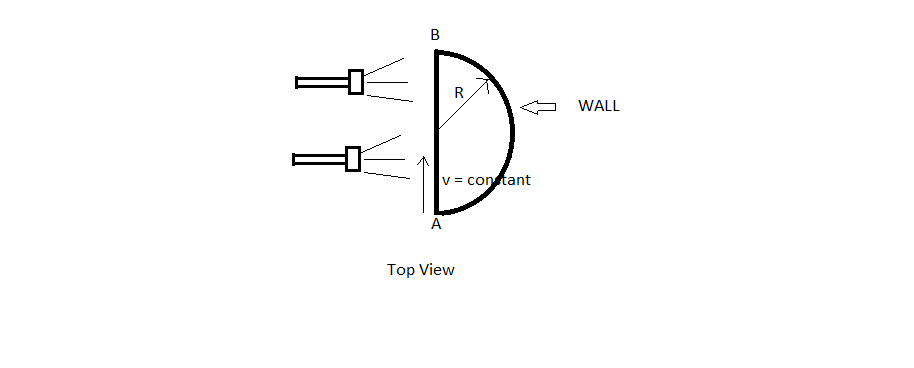A small block can move in a straight horizontal line $AB$.Flash lights from one side projects its shadow on a curved vertical wall which has a horizontal cross section as a circle. If tangential and normal acceleration of shadow of the block on the wall as a function of time can be represented as:

$a_N=\frac{cvR}{(2Rt-vt^2)} \quad , \quad a_T=\frac{R(vt-R)v^{\frac{d}{e}}}{(2Rt-vt^2)^{\frac{f}{g}}}$.

Find $|c|+|d|+|e|+|f|+|g|$

Details and Assumptions

• As shown in figure $v$ is the constant along $AB$.

• Given Figure is top view of the setup.

• $c,d,e,f,g$ are natural numbers and $\frac{d}{e} ,\frac{f}{g}$ are in simplest forms.

• $a_N,a_T$ represent normal and tangential accelerations respectively.